# How to Average the Absolute Values in Excel?

Sometimes, in Excel, you want to calculate the average of absolute values. If we consider the values as positive, even if they are negative, then they are called the absolute values. The absolute values are always positive. Basically, absolute values are used in calculating distances or inequalities. If we want to calculate the average distance travelled by a delivery person in a day, we can use this process. This tutorial will help you understand how we calculate averages for absolute values in Excel.

## Average Absolute Values in Excel with Helper Column

Here, we will first use the ABS formula and then the AVERAGE formula to get our average. Let us see a straightforward process to understand how we can calculate the average for absolute values using a helper column in Excel.

Step 1

Let us consider an Excel sheet that contains a list of numbers, as shown in the below image.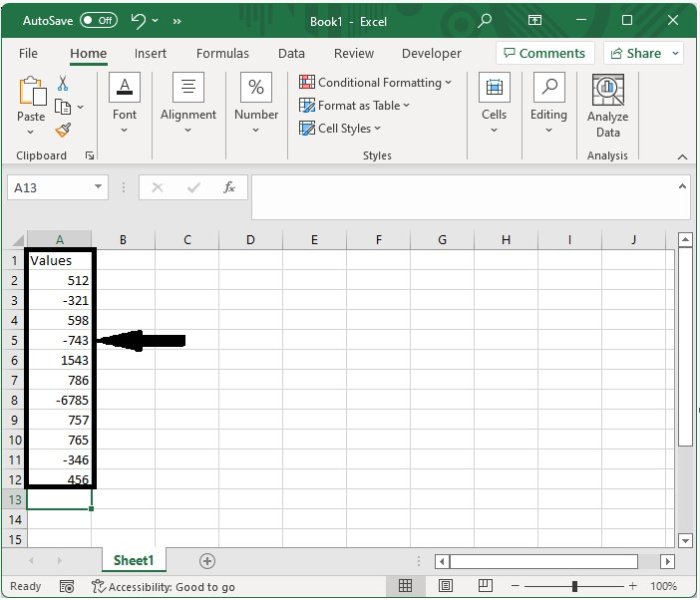Now we need to convert the values to absolute values. Click on an empty cell, type the formula as =ABS(A2), and click on OK to get the first value.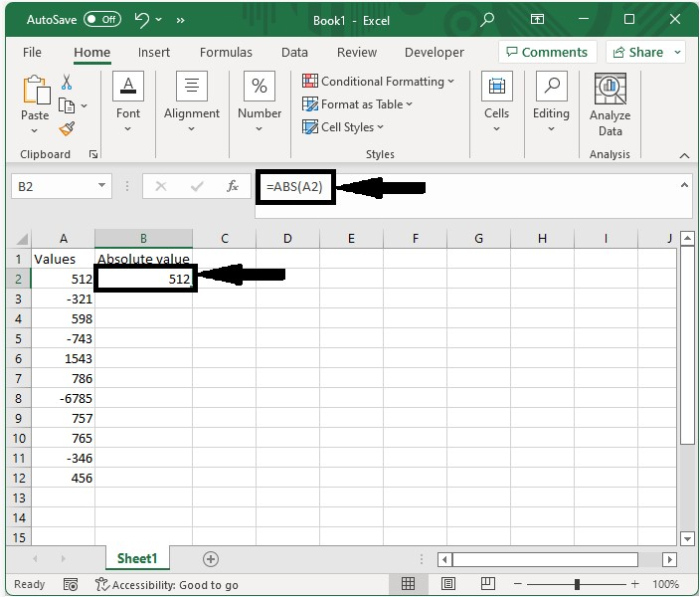Step 2

Drag down using the auto-fill handle to get all the other results, as shown in the below image.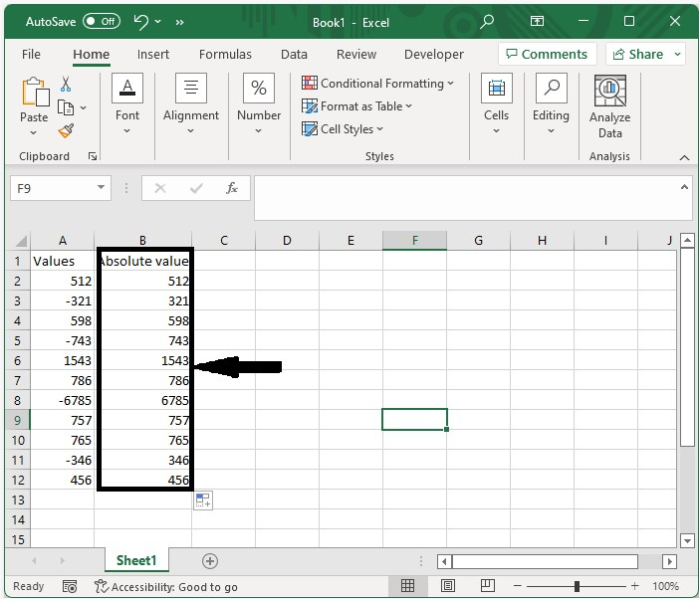Step 3

To get the average, click on an empty cell, type the formula =AVERAGE(B2:B12), and then click OK.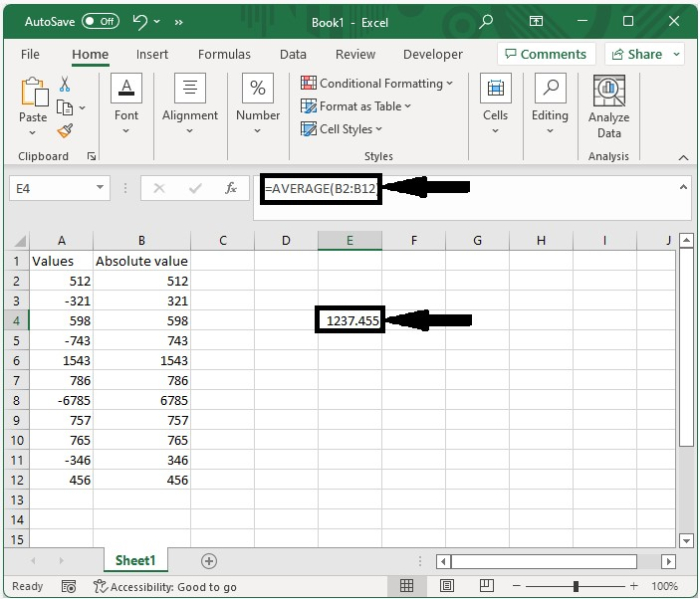## Average Absolute Values with Formula

Here we will combine both the ABS and average formulas into a single formula. Let's see how we can calculate the average for absolute values in Excel with a formula.

Let us consider the same data that we used in the above example and click on an empty cell and type the formula =AVERAGE(ABS(A2:A12)) directly in the formula to get the result shown in the below image.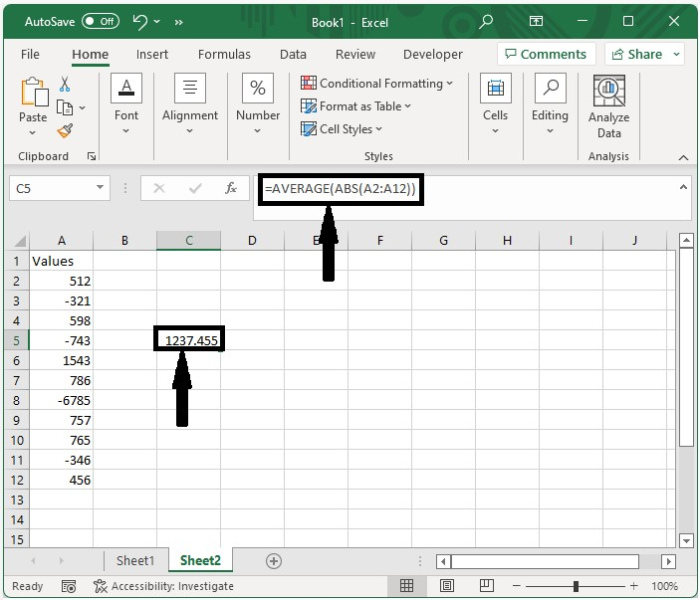## Conclusion

In this tutorial, we used a simple example to demonstrate how we can calculate the average for absolute values in Excel.

Updated on: 12-Jan-2023

1K+ Views